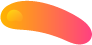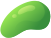##### Find the present value of annuity

An annuity pays 600 at the end of each month over a period of four
years. Interest is the first year is a nominal 8% convertible monthly. Interest in
the second year is a nominal 5% convertible monthly. Interest in the third year
is a nominal 7.55% convertible monthly. Interest in the final year is nominal 12% convertible monthly. Each of these rates applies during its respective year for all payments. Find the present value of this annuity at inception.

A twenty-year annuity-immediate pays 5000 the first year and increases
by X each year thereafter. The interest rate is 6.5% effective annual. If the present value of this annuity is 70183.23, find the value of X